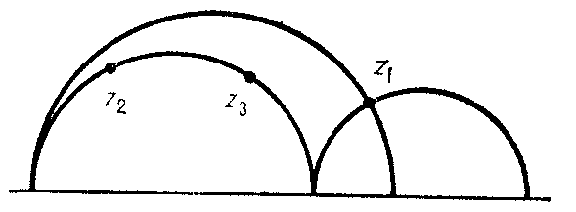# Poincaré model

(diff) ← Older revision | Latest revision (diff) | Newer revision → (diff)

Poincaré interpretation

A model realizing the geometry of the Lobachevskii plane (hyperbolic geometry) in the complex plane. In the Poincaré model with a circular absolute, every point of the unit disc $E=\{z\colon|z|<1\}$ in the complex plane is called a hyperbolic point and the disc itself is called the hyperbolic plane. Circular arcs (and diameters) in $E$ which are orthogonal to the boundary circle $\Omega=\{z\colon|z|=1\}$ are called hyperbolic lines. Every point of $\Omega$ is called an ideal point. Hyperbolic lines with a common hyperbolic point are said to be intersecting lines; those with a common ideal point are called parallel; and those lines which do not intersect and are not parallel are called ultra-parallel (divergent). So, for example, in Fig. a two lines are depicted passing through the point $z_1$ and both are parallel to the line $z_2z_3$.Figure: p073070a

In the Poincaré model in the half-plane $H=\{z=x+iy\colon y>0\}$, every point of the upper half-plane is called a hyperbolic point and the half-plane itself is called the hyperbolic plane. Semi-circles and half-lines which are orthogonal to the real axis are called hyperbolic lines. The set of ideal points (the absolute) is the real axis together with the point at infinity of the $z$-plane. Parallel, intersecting and divergent lines are defined as in the Poincaré model with a circular absolute. So, for example, in Fig. b two lines passing through the point $z_1$ and parallel to the line $z_2z_3$ are depicted.Figure: p073070b

The motions can be described as conformal transformations sending the absolute onto itself. Distance is defined using the cross ratio of four points:

$$\rho(z_1,z_2)=k\ln(z_1,z_2,z_1^*,z_2^*),$$

where $z_1^*$ is the ideal point of the half-line emanating from $z_1$ and passing through $z_2$, $z_2^*$ is the ideal point of the half-line emanating from $z_2$ and passing through $z_1$, $k$ is an arbitrary positive constant, and

$$(z_1,z_2,z_1^*,z_2^*)=\frac{z_1^*-z_1}{z_1^*-z_2}:\frac{z_2^*-z_1}{z_2^*-z_2}$$

The angular measurements in the Poincaré model are the same as in hyperbolic geometry (cf. Lobachevskii geometry).

H. Poincaré (1882) proposed this model.

How to Cite This Entry:
Poincaré model. Encyclopedia of Mathematics. URL: http://encyclopediaofmath.org/index.php?title=Poincar%C3%A9_model&oldid=32936
This article was adapted from an original article by A.B. Ivanov (originator), which appeared in Encyclopedia of Mathematics - ISBN 1402006098. See original article